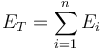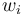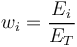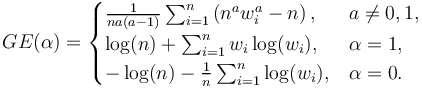# Generalized Entropy Index

## Definition

For the purpose of measuring concentration, the Generalized Entropy Index is measure of concentration that draws from concepts of information theory

## Details

If the total exposure is$E_T = \sum^{n}_{i=1} E_{i}$

and the fractional exposures$w_i$ are defined as$w_{i} = \frac{E_i}{E_T}$

Then the Generalized Entropy index is defined as$GE(\alpha) = \begin{cases} \frac{1}{n a (a-1)} \sum_{i=1}^n \left( n^a w_i^a -n \right),& a \ne 0, 1,\\ \log(n) + \sum_{i=1}^n w_i \log( w_i) ,& \alpha=1,\\ - \log(n) - \frac{1}{n} \sum_{i=1}^n \log( w_i) ,& \alpha=0. \end{cases}$

### Relation with the Theil Index

The Theil Index is the Generalized Entropy Index for a = 1

None

None

None

## Implementations

Open Source implementations of the Generalized Entropy index are available in